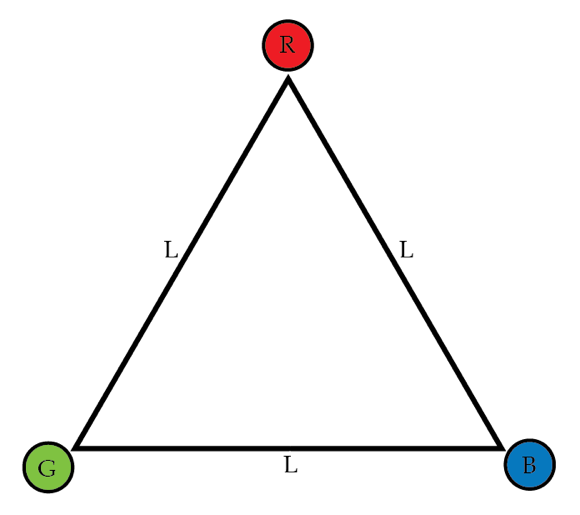# Don't forget the 3rd law

Newton's third law, that for every action there is an equal and opposite reaction, is essentially a statement about conservation. Without it, we wouldn't have the conservation of energy, momentum, or angular momentum.

We can see this with a simple example: Imagine we have two equal mass objects, A and B, separated by some distance $x$. A feels an attractive force towards B with some strength F. If we have Newton's third law, then that means that B would be attracted to A by a force of the same strength $F$. Imagine now we violate this law, and say that A is attracted to B but B is repelled from A (so the forces are in the same direction). Then the AB pair of objects would zoom off to infinity, thereby violating momentum conservation.

We can play a similar game with angular momentum. I have three objects labelled, (R)ed, (G)reen, and (B)lue each with mass $1~\mbox{g}$. The magnitude of the forces between the objects follows an inverse force law, i.e.

$|F|=\frac{1}{d}$ Newtons

where $d$ is the distance between the objects in meters. Such a force, while a little different than you are used to, occurs in nature, for example in 2-d systems. However, I am now going to violate Newton's third law, and give the following rules for how R,G, and B attract or repel:

R is attracted to G, but G is repelled from R.

G is attracted to B, but B is repelled from G.

B is attracted to R, but R is repelled from B.

I arrange my three objects so they are at rest at the tips of an equilateral triangle of side length $L$ meters. At $t=0$ I let go of the objects. After $5~\mbox{seconds}$, what is the change in the total angular momentum of the system about the centroid of the triangle in $\mbox{kg}~\mbox{m}^2/\mbox{s}\,$?×

Problem Loading...

Note Loading...

Set Loading...